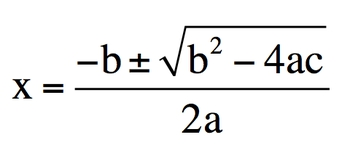## GOB Chemistry

Learn the toughest concepts covered in your GOB - General, Organic, and Biological Chemistry class with step-by-step video tutorials and practice problems.

BONUS: Mathematical Operations and Functions

The quadratic formula can be used to solve for the variable x when given an algebraic equation in the form of:ax2 + bx - c.

The quadratic formula is most commonly used for questions dealing with chemical equilibrium where you have to use an ICE Chart

1
concept